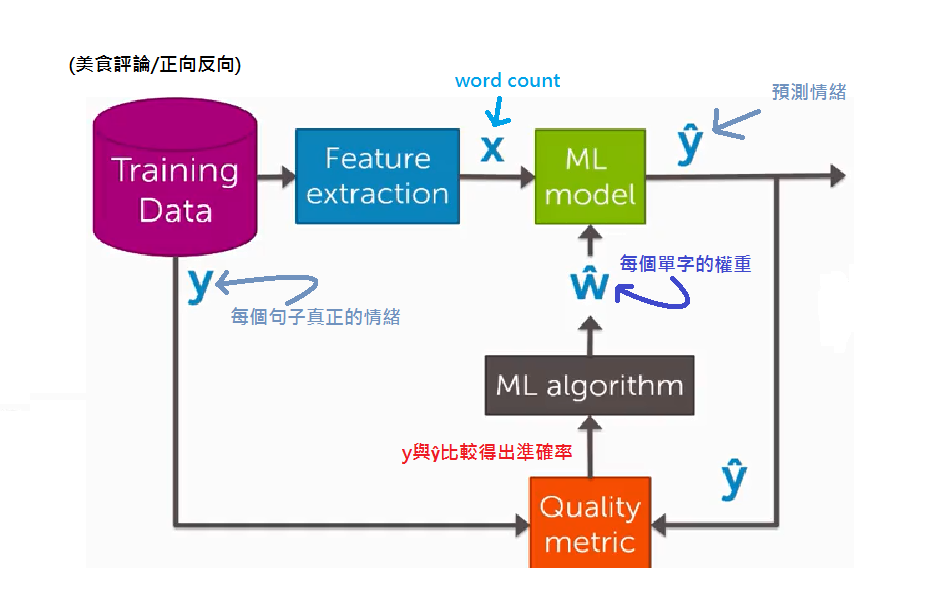#2018 iT 邦幫忙鐵人賽DAY 8
0
AI & Machine Learning

# Classifictaion

## False positives, false negatives, and confusion matrices

classifiers做出的預測為Predicted label而真實的結果為True label，在這樣的情境下會有四種的結果

Predicted label + Predicted label -
True label + True Positive False Negative
True label - False Negativ True Negativ
• False negative︰classifiers預測為False，真實結果為True
• False positive︰classifiers預測為True，真實結果為False

• 垃圾郵件過濾
• False negative︰要動手清除郵件，有點煩躁
• False positive︰這代表你有一封相對重要信件有可能永遠看不到了
• 醫療診斷
• False negative︰我的病沒有被診斷出來，嚴重的話可能會導致延誤就醫
• False positive︰我沒病卻判定有病，我可能要吃一堆根本沒用的藥，然後承受那些藥的副作用

-正確率=(60+12+8)/100 = 80%

## Class probabilities

-味增湯很好喝，服務品質優良 <- ""+""
-味增湯好喝，涼麵普通 <-不確定

-味增湯很好喝，服務品質優良 <- P(y=+|x) = 0.99 //正面機率為0.99
-味增湯好喝，涼麵普通 <- P(y=+|x) = 0.55 //負面機率為0.55，因為我們不是很確定

## Classification ML block diagram• 每一個評論都帶有情緒標籤(正/負)
• 我們針對評論進行一次特徵提取
• 每一個評論都可以得到一個單字計數x
• 若有其他參數則稱為w-hat，在本章節中常用是單字的權重(好吃2分/難吃-2.5...)
• 接著從ML model可以得到ŷ(我們的預測結果)，當它大於0時為正向，反之亦然
• 再來我們將每一次的測試結果ŷ與y(測試集)做比較
• 最後將分類的正確率餵給 ML algorithm，讓他來提高我的正確率